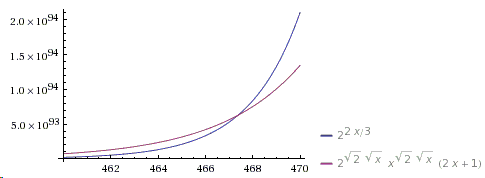# Bertrand's Postulate - Paul Ërdos' First Published Paper

If there was competition for the most misnamed math fact, "Bertrand's Postulate" would be certainly among the very top contenders. The statement of the "postulate" was conjectured in 1845 by Joseph Bertrand and first proved by Pafnuty Chebyshev in 1850. So, between 1845 and 1850 it should have been called a conjecture, and afterwards a theorem (which is how it is sometimes being referred to.) The word "postulate" has probably stuck with it because Bertrand had verified its validity empirically for all $n\lt 3000000$. But what is this theorem? There are two slightly different formulations:

For every $n\gt 1$, there is some prime $p$ with $n\lt p\lt 2n$.

Or, equivalently,

For every $n\ge 1$, there is some prime $p$ with $n\lt p\le 2n$.

The theorem has been also proved by the famous Indian mathematician Ramanujan and in 1932 by Paul Ërdos when he was only 19 years old. This was Ërdos' - who in time became the most prolific mathematician of all times - the very first paper. Ërdos used bounds on the magnitude of the central binomial coefficient to prove the statement for $l\ge 4000,$ leaving the smaller values for manual (or nowadays computer) verification. Even without computers that was not a big deal. With an attribution to Edmund Landau, Ërdos produced a sequence of primes

$2,3,5,7,13,23,43,83,163,317,631,1259,2503,4001,$

in which each number is smaller than twice its predecessor. Thus in any interval $n,2n$, with $n\le 4000$, there is bound to be a prime - a member from the sequence.

Several books I came across follow Ërdos' original ideas. To the extent I can judge (I do not read German), Aigner and Ziegler give a most faithful presentation of Ërdos' proof, although, as they claim, of not the original one. In Underwood Dudley's variant, the reader is required to verify the theorem for $n\le 2787$ while Victor Moll leaves for manual verification only $n\le 467$.

### Lemma 1

For all integer $n\gt 0$,

$\displaystyle {2n \choose n} \ge \frac{4^n}{2n+1}$

### Proof

$\displaystyle{2n \choose n}$ is the central binomial coefficient: $\displaystyle{2n \choose n}=\frac{(2n)!}{n!n!}$, it's the largest of all coefficients in the expansion of $\displaystyle(1+1)^{2n}=\sum_{k=0}^{2n}{2n \choose k}$. This shows that $\displaystyle4^{n}=2^{2n}\le (2n+1){2n \choose n}$.

The next step is to determine the $p$-adic valuation of $\displaystyle{2n \choose n}$. This will be based on the following property of the valuation:

### Lemma 2

For integer $a,b$

$\nu_{p}(a\cdot b)=\nu_{p}(a) + \nu_{p}(b)$ and
$\nu_{p}(\displaystyle\frac{a}{b})=\nu_{p}(a) - \nu_{p}(b)$.

### Lemma 3

If $\displaystyle\frac{2n}{3}\lt p \le n$, then $\displaystyle\nu_{p}({2n \choose n}) = 0.$

In other words, $p$ does not divide $\displaystyle {2n \choose n}$.

### Proof

If $\displaystyle\frac{2n}{3}\lt p \le n$, $(2n)!$ has two factors of $p$ - one coming from $p$, the other from $2p$, while $\nu_{p}(n!)$ has exactly one factor.

As a consequence of Lemma 2,

$\nu_{p}({2n \choose n}) = \nu_{p}((2n)!)-2\nu_{p}(n!) = 2 - 2\cdot 1 = 0$.

### Lemma 4

If $p$ divides $\displaystyle{2n \choose n}$, then $\displaystyle\nu_{p}({2n \choose n})\le\mbox{log}_{p}(2n)$.

### Proof

Define $r(p)$ by the inequalities $p^{r(p)}\le 2n \lt p^{r(p)+1}$. Then, like in the proof of Legendre's Theorem,

\displaystyle \begin{align} \nu_{p}({2n \choose n}) &= \nu_{p}((2n)!)-2\nu_{p}(n!) \\ &= \sum_{i=1}^{r(p)}\left( \Big\lfloor\frac{2n}{p^i}\Big\rfloor - 2\Big\lfloor\frac{n}{p^i}\Big\rfloor \right) \\ &\le r(p), \end{align}

because, for $x\in\mathbb{R}$, $\lfloor 2x\rfloor - 2\lfloor x\rfloor$ is either $0$ or $1$.

### Lemma 5

For $n\in\mathbb{N}$ and the product running over primes:

$\displaystyle \prod_{p\le n}p\le 4^n.$

### Proof

The argument is by induction on $n$. For $n=1$, the claim follows by definition of an "empty" product: $\displaystyle \prod_{p\le 1}p = 1\le 4$. For $n=2$, it reduces to $2\le 4^2$. For even $n\ge 4$, by induction

$\displaystyle \prod_{p\le n}p = \prod_{p\le n-1}p \le 4^{n-1}\lt 4^n.$

For odd $n$, say $n=2m+1$,

$\displaystyle \prod_{p\le n}p = \prod_{p\le m+1}p\times \prod_{p\le m+2}^{2m+1}p \le 4^{m+1}{2m+1 \choose m},$

where the first product is bounded by the inductive hypothesis, while the bound for the second product stems from the fact that $\displaystyle{2m+1 \choose m}$ is divisible by every prime $p$ in the range $m+2\le p\le 2m+1$. The required inequality then follows from $\displaystyle{2m+1 \choose m}\le 2^{2m}$. The latter follows from the binomial theorem:

$\displaystyle 2{2m+1 \choose m} = {2m+1 \choose m} + {2m+1 \choose m+1} \le \sum_{i=0}^{2m+1}{2m+1 \choose i} = (1+1)^{2m+1}.$

### Proof of Bertrand's Postulate

Assume that, for some $n$, there is no prime $p$, $n\lt p\le 2n$. The central binomial coefficient ${2n \choose n}$ has at most $\sqrt{2n}$ prime factors smaller than $\sqrt{2n}$. The contribution of each of these factors to ${2n \choose n}$ does not exceed $2n$. On the other hand, by Lemma 4, every prime $p\ge\sqrt{2n}$ satisfies $\nu_{p}({2n \choose n})\le 1$. Combining these gives

\displaystyle \begin{align} {2n\choose n}&\le (2n)^{\sqrt{2n}}\prod_{\sqrt{2n}\lt p\le 2n/3}p \\ &\le (2n)^{\sqrt{2n}}\prod_{p=2}^{2n/3}p \\ &\le (2n)^{\sqrt{2n}}4^{2n/3}. \end{align}

Together with Lemma 1, this leads to

$\displaystyle \frac{4^n}{2n+1}\le (2n)^{\sqrt{2n}}4^{2n/3},$

which may be rewritten as

$\displaystyle 4^{n/3}\le (2n+1)(2n)^{\sqrt{2n}}.$

This inequality fails for $n\ge 468$:As was mentioned at the beginning, Bertrand's Postulate can be manually verified for $n\le 467$.

### Reference

1. M. Aigner, G. Ziegler, Proofs from THE BOOK, Springer, 2000, 7-13
2. U. Dudley, Elementary Number Theory, Dover, 2008, 177-179
3. P. Ërdos, Beweis eines Satzes von Tschebyshef, Acta Sci. Math (Szeged) 5 (1930-1932), 194-198 (in German)
4. R. Graham, D. Knuth, O. Patashnik, Concrete Mathematics, 2nd edition, Addison-Wesley, 1994
5. V. H. Moll, Numbers and Functions: From a Classical-Experimental Mathematician's Point of View, AMS, 2012, 89-91Copyright © 1996-2018 Alexander Bogomolny

68557968